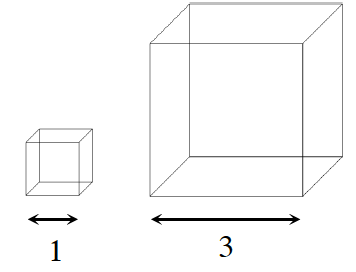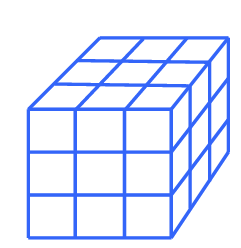### Home > CCG > Chapter Ch8 > Lesson 8.3.3 > Problem8-122

8-122.

Multiple Choice: How many cubes with edge length $1$ unit would fit in a cube with edge length $3$ units? Homework Help ✎

1. $3$

1. $9$

1. $10$

1. $27$

1. None of theseDraw a rough sketch of a 3 unit cube with 1 unit cubes inside it.

Multiply (length)(width)(height) to determine the correct answer.